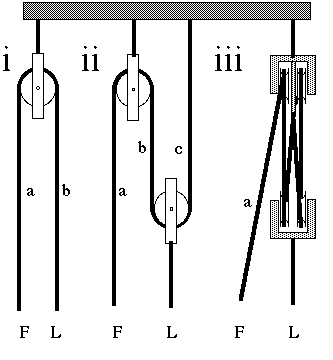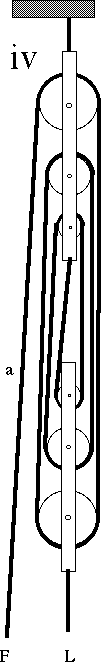## The physics of blocks and pulleys

 Diagram (i) shows a pulley being used just to change the direction of a force. You can pull on the end of rope marked a with force F and the end b moves an equal distance with load force L (neglecting friction). (ii) shows an arrangement that doubles the force. You pull with force F on the section a. Again neglecting friction, the sections of the same rope marked b and c are also under tension F, so the second block is pulled up by 2F, so the load lifted L = 2F. This extra force does not come 'free', however. When you pull a distance x on a, x is the total amount of shortening in both b and c, so each of b and c is shortened by x/2, which is how much the load L rises. The work you do is Fx, the work done on the load is L.x/2 = Fx. In (iii), pulling on a causes four sections of the rope to shorten and to share the load. So these blocks multiply the force by four times, but you have to pull 4 times as far.In iv, there are six sections of rope. So these blocks multiply the force by six times, but you have to pull 6 times as far. The diagrams show the blocks in arrangements suitable for lifting. For the main sheet on a boat (the rope that controls the mainsail), systems like (iii) and (iv) are used, but they are mounted the other way up, so that the end upon which you pull is at the traveller on deck. Usually there is a cleat: a system for holding the main sheet in fixed position once the sail is set.This work is licensed under a Creative Commons License.